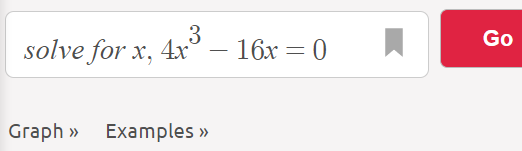# How to Maximize a Function

## How to Maximize a Function: General Steps

General steps to maximize a function on a closed interval [a, b]:

1. Find the first derivative,
2. Set the derivative equal to zero and solve,
3. Identify any values from Step 2 that are in [a, b],
4. Add the endpoints of the interval to the list,
5. Evaluate your answers from Step 4: The largest function value is the maximum.

Example problem #1: Find the maximum of the function f(x) = x4 – 8x2 + 3 on the interval [-1, 3].

Step 1: Find the first derivative. Our function is f(x) = x4 – 8x2 + 3, so we can take the derivative with the power rule, giving:
f′(x) = 4x3 – 16x.

Step 2: Set the derivative equal to zero and solve, using algebra.

• 4x3 – 16x = 0.
• x3 – 4x = 0 (Dividing by 4).
• x(x – 2)(x + 2) = 0((Factoring)

Solving, the critical points are -2, 0 and 2.

If algebra isn’t your strong point, use Symbolab’s algebra calculator to solve for 0. Here’s the format:Step 3: Identify any values from Step 2 that are in [a, b]. These values are all potential maximums and are called critical points.

The interval given in the question was [-1, 3]. Of the three critical points we found: -2, 0 and 2, only two are in the specified interval: 0 and 2.

Step 4: Add the endpoints of the interval to the list.
The endpoints of the interval are given in the question as -1 and 3, which gives a total of four possibilities: -1, 0, 2, and 3.

Step 5: Plug the values from Step 4 into the original function (if you have a TI-89, creating a table of values makes this step a lot faster!):

• f(-1) = -14 – 8(-1)2 + 3 = -4,
• f(0) = 04 – 8(0)2 + 3 = 3,
• f(2) = 24 – 8(2)2 + 3 = -13,
• f(3) = 34 – 8(3)2 + 2 = 12.

The largest function value is the maximum; The function has a maximum at x = 3 on the interval [-1, 3].

We can confirm this with a graph (I used Desmos.com):